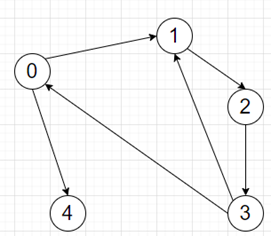# Program to find shortest cycle length holding target in python

Suppose we have adjacency list of a directed graph, where each list at index i is represented connected nodes from node i. We also have a target value. We have to find the length of a shortest cycle that contains the target. If there is no solution return -1.

So, if the input is liketarget = 3., then the output will be 3, as the cycle is nodes 1 -> 2 -> 3 -> 1. Note there is another cycle 0 -> 1 -> 2 -> 3 -> 0, but this is not shortest.

To solve this, we will follow these steps -

• visited := a new set
• l := a list with element target.
• length := 0
• while l is non-empty, do
• length := length + 1
• nl := a new list
• for each u in l, do
• for each v in graph[u], do
• if v is same as target, then
• return length
• if v is visited, then
• go for the next iteration
• mark v as visited
• insert v at the end of nl
• l := nl
• return -1

Let us see the following implementation to get better understanding -

## Example

Live Demo

class Solution:
def solve(self, graph, target):
visited = set()
l = [target]
length = 0

while l:
length += 1
nl = []
for u in l:
for v in graph[u]:
if v == target:
return length
if v in visited:
continue
nl.append(v)
l = nl

return -1

ob = Solution()
graph = [[1, 4],,,[0, 1],[]]
target = 3
print(ob.solve(graph, target))

## Input

[[1, 4],,,[0, 1],[]]

## Output

3